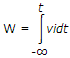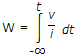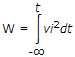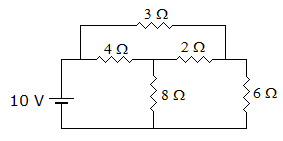# Electronics and Communication Engineering - Networks Analysis and Synthesis

1.

Which of the following is correct?

 A. Voltages across a capacitance can change instantaneously B. Voltages across a capacitance cannot change instantaneously C. Charge across a capacitance is always constant D. Charge across a capacitance can change instantaneously

Explanation:

No answer description available for this question. Let us discuss.

2.

The V-i relation for a varistor is

 A. i = kvn B. v = kin C. i = kv3 D. v = ki3

Explanation:

No answer description available for this question. Let us discuss.

3.

For 2 port reciprocal network, o.c. output voltage divided by input current is equal to

 A. B B. z12 C. 1/y12 D. h12

Explanation:

No answer description available for this question. Let us discuss.

4.

The energy dissipated W in a resistance having voltages v and current i is given by

 A.B. W = vit2 C.D.Explanation:

No answer description available for this question. Let us discuss.

5.

In figure, the current through 2 Ω resistance isA. zero B. -2A C. 2A D. 1A